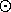Plethysms

## Plethysms

An important multiplication (introduced by Littlewood) of characters, Schur polynomials or polynomials in general is that of plethysm. SYMMETRICA contains an efficient routine concerning specific plethysms of symmetric polynomials which is due to C. Carré. Here are the main lines of an example program:
```complete_schur_plet(n,a,b);println(b);
elementary_schur_plet(n,a,c);println(c);
l_plet_complete_schur(l,n,a,d);println(d);
l_plet_elementary_schur(l,n,a,e);println(e);
```
The output gives the decomposition of the representations
[n][a], [1n][a]
in the notation of James/Kerber, or of
{n}Ä{a}, {1n}Ä{a}
in terms of Schur polynomials and in the notation of Littlewood. (Recall that the complete symmetric polynomial is the Schur polynomial corresponding to the identity representation [n] while the elementary symmetric polynomial corresponds to the alternating representation.) The routines l_plet* evaluate the irreducible constituents with length (=number of parts) £l. If you enter l=n=2 together with the partition a=1 2, then you obtain the following output:

1 1113 1 123 1 222 1 24

1 1122 1 114 1 123 1 33

1 24

1 33

This shows, for example, that[2,1]=[3,13]+[3,2,1]++[4,2]
(in the notation of James/Kerber, while the above output uses the French notation).
harald.fripertinger@kfunigraz.ac.at,
last changed: November 19, 2001Plethysms